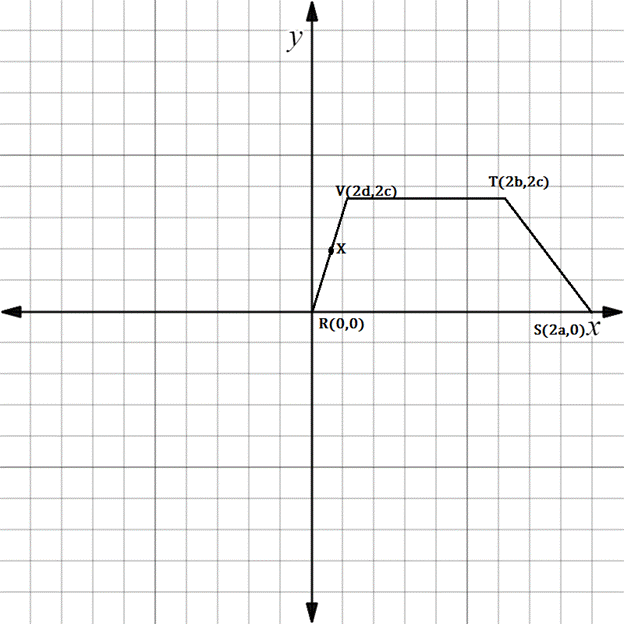Chapter 10.3, Problem 32E### Elementary Geometry for College St...

6th Edition
Daniel C. Alexander + 1 other
ISBN: 9781285195698

#### Solutions

Chapter
Section### Elementary Geometry for College St...

6th Edition
Daniel C. Alexander + 1 other
ISBN: 9781285195698
Textbook Problem
1 views

# Which formula would you use to establish each of the following claims?a) The coordinates of X are d ,   c .b) m V T - = 0 c) V T - ║ R S - d) The length of R V - is 2 d 2 + c 2Trapezoid R S T V ; X is the midpoint of R V - .

To determine

(a)

To find:

The formula used to find the coordinates of X.

Explanation

Since X is found midway between R and V.

Hence, midpoint formula is used

To determine

(b)

To find:

The formula used to establish mVT-=0.

To determine

(c)

To find:

The formula used to establish VT-RS-.

To determine

(d)

To find:

The formula used to establish the length of RV- is 2d2+c2.

### Still sussing out bartleby?

Check out a sample textbook solution.

See a sample solution

#### The Solution to Your Study Problems

Bartleby provides explanations to thousands of textbook problems written by our experts, many with advanced degrees!

Get Started

#### Differentiate the function. f(x)=ln1x

Single Variable Calculus: Early Transcendentals, Volume I

#### In problems 33-36, write an inequality that discribes each interval or graph. 36.

Mathematical Applications for the Management, Life, and Social Sciences

#### limh0ah1h=1 for a = a) 0 b) 1 c) e d) The limit is never 1.

Study Guide for Stewart's Single Variable Calculus: Early Transcendentals, 8th